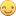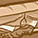## Genetic Algorithm For Integer Programming - Football Players Selection

Started ‎02-15-2015 by
Modified ‎04-04-2022 by
Views 1,753

Genetic Algorithm  is so sharp and powerful and almost be able to solve all kind of optimum problem.GA is a such powerful tool, you don't need OR any more. Just kidding.

Which means You can solve all the optimum problems without any money if you have SAS University Edition.Code is written in IML.

It is so amazing . I love it so much.

https://communities.sas.com/message/256779#256779

data have;

input Player Position \$  Points Price ;

cards;

1 G 2 8000

2 D 4 7000

3 M 6 12000

4 A 8 10000

5 G 4 9000

6 A 9 14000

8 D 4 8000

9 M 6 4000

10 A 8 10000

12 A 9 14000

13 D 4 7000

14 M 6 12000

17 A 9 14000

18 G 2 8000

19 D 4 7000

20 M 6 12000

24 D 4 7000

25 M 6 12000

29 D 4 7000

30 M 6 7000

32 M 6 1000

36 D 4 7000

37 M 6 12000

42 D 4 7000

43 M 6 12000

45 G 4 9000

46 A 9 14000

47 D 4 7000

48 M 6 12000

55 D 4 7000

56 M 6 12000

60 D 4 6000

61 M 6 9000

63 M 6 2000

67 D 4 5000

68 M 6 9000

70 M 8 6000

;

run;

%macro createData(n=2);

data have(drop=i rename=(count=player));

set have(drop=player);

do i = 1 to &n;

count+1;

output;

end;

run;

%mend createData;

%createData()

options fullstimer;

proc sort data=have;by Position Points ;run;

proc sql noprint;

select count(*) into : A from have where Position='A';

select count(*) into : D from have where Position='D';

select count(*) into : G from have where Position='G';

select count(*) into : M from have where Position='M';

quit;

proc iml;

use have;

Player_A=Player;Point_A=Points;Pice_A=Price;

Player_D=Player;Point_D=Points;Pice_D=Price;

Player_G=Player;Point_G=Points;Pice_G=Price;

Player_M=Player;Point_M=Points;Pice_M=Price;

close have;

start football(x) global(Point_A,Pice_A,Point_D,Pice_D,Point_G,Pice_G,Point_M,Pice_M);

points=sum(Point_A[x[1:2]],Point_D[x[3:6]],Point_G[x],Point_M[x[8:11]]);

sum_cost=sum(Pice_A[x[1:2]],Pice_D[x[3:6]],Pice_G[x],Pice_M[x[8:11]]);

if sum_cost>75000 | ncol(unique(x[8:11]))^=4  | ncol(unique(x[3:6]))^=4 | ncol(unique(x[1:2]))^=2  then points=1;

return (points);

finish;

id=gasetup(2,11,123);

call gasetobj(id,1,"football");

call gasetcro(id,1.0,2);

call gasetmut(id,0.2,2,1);

call gasetsel(id,100,1,0.95);

call gainit(id,10000,{1  1  1  1  1  1  1  1  1  1  1 ,

&A &A &D &D &D &D &G &M &M &M &M });

niter = 100;

summary = j(niter,2);

mattrib summary [c = {"Max Points", "Avg Points"} l=""];

do i = 1 to niter;

call garegen(id);

call gagetval(value, id);

summary[i,1] = value;

summary[i,2] = value[:];

end;

call gagetmem(mem, value, id, 1);

Players=Player_A[mem[1:2]]//Player_D[mem[3:6]]//Player_G[mem]//Player_M[mem[8:11]];

sum_cost=sum(Pice_A[mem[1:2]],Pice_D[mem[3:6]],Pice_G[mem],Pice_M[mem[8:11]]);

print Players[ l="best member:"],

"Max Points: " value[l = ""],

"Total Cost:  " sum_cost[l = ""] ;

iteration = t(1:niter);

print iteration summary;

call gaend(id);

quit;Xia KeshanDoes this mean you can write the optimal march madness bracket?Yeah. I bet it was . If you read the IML documentation about GA . You would be shocked by it . It almost can solve all the optimize problem .But you need to know how to make it fast convergence . There are so many parameters you need to set up . Once you master GA , you maybe not need OR any more .  I don't know what Mr. Newton  would get to say , if he was still live today .Nice @Ksharp

But why Do yo duplicate the data when doing:

%macro createData(n=2);

data have(drop=i rename=(count=player));

set have(drop=player);

do i = 1 to &n;

count+1;

output;

end;

run;

%mend createData;

If I change into (n=1) it's still working. I don't the the need for it but probably I'm missing out something.

Best regards, ArneYeah. That is just creating a sample data. any value of n is validate .

Version history
Last update:
‎04-04-2022 03:12 PM
Updated by:Contributors
Article Labels